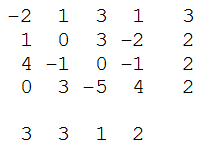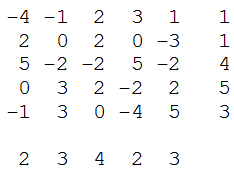# Self-Descriptive Squares

Lee Sallows has been working on a new experiment in self-reference that he calls self-descriptive squares, arrays of numbers that inventory their own contents. Here’s an example of a 4×4 square:The sums of the rows and columns are listed to the right and below the square. These sums also tally the number of times that each row’s rightmost entry, or each column’s lowermost entry, appears in the square. So, for example, the sum of the top row is 3, and that row’s rightmost entry is 1; correspondingly, the number 1 appears three times in the square. Likewise, the sum of the rightmost column is 2, and the lowermost entry in that column, 4, appears twice in the square.

In this example this property extends to the diagonals — and, pleasingly, each sum applies to both ends of its diagonal. The northwest-southeast diagonal totals 2, and both -2 and 4 appear twice in the square. And the southwest-northeast diagonal totals 3, and both 1 and 0 appear three times.

“Easy to understand, but not so easy to produce!” he writes. “I’m still in the throes of figuring out the surprisingly complicated theory of such squares. It turns out there are just two basic squares of 3×3. One of them can be found at the centre of this 5×5 example, which is therefore a concentric self-descriptive square:”(Thanks, Lee.)